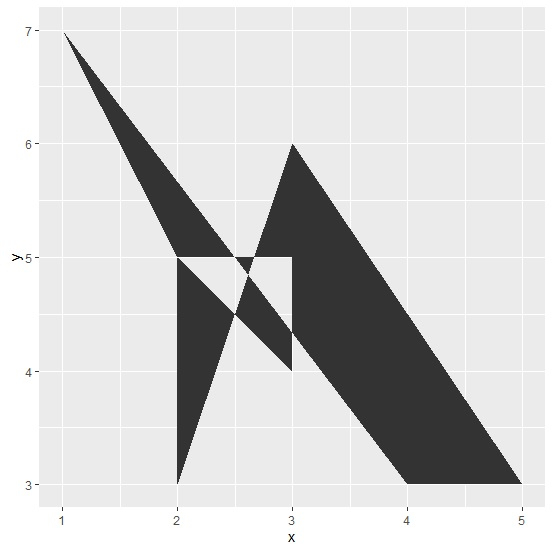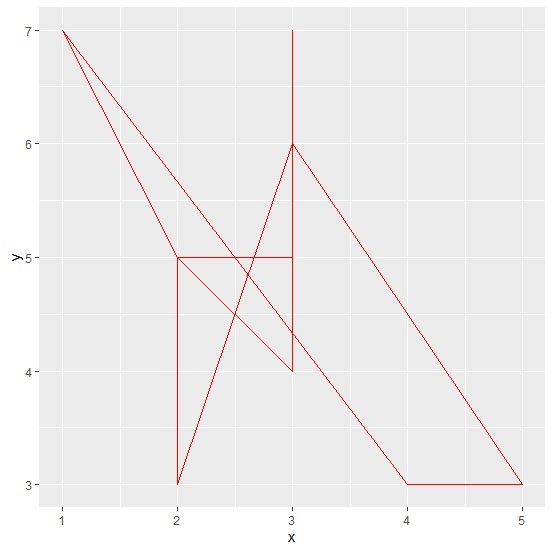# How to create a transparent polygon using ggplot2 in R?

A transparent polygon just represents the border lines and a hollow area; thus, we can only understand the area covered but it becomes a little difficult to understand the scales. Hence, this visualisation technique is not as useful as others that fills the area with a different color. But it could be used if the range of the data is not large.

Consider the below data frame −

## Example

Live Demo

set.seed(123)
x<-sample(1:5,10,replace=TRUE)
y<-sample(3:8,10,replace=TRUE)
df<-data.frame(x,y)
df

## Output

   x y
1  2 8
2  4 5
3  3 7
4  5 6
5  5 3
6  1 8
7  3 4
8  5 3
9  3 4
10 3 8

Loading ggplot2 package and creating a polygon using x and y −

## Example

library(ggplot2) ggplot(df,aes(x,y))+geom_polygon()

## OutputCreating a polygon using x and y with transparency −

## Example

ggplot(df,aes(x,y))+geom_polygon(fill=NA,color="red")

## Output